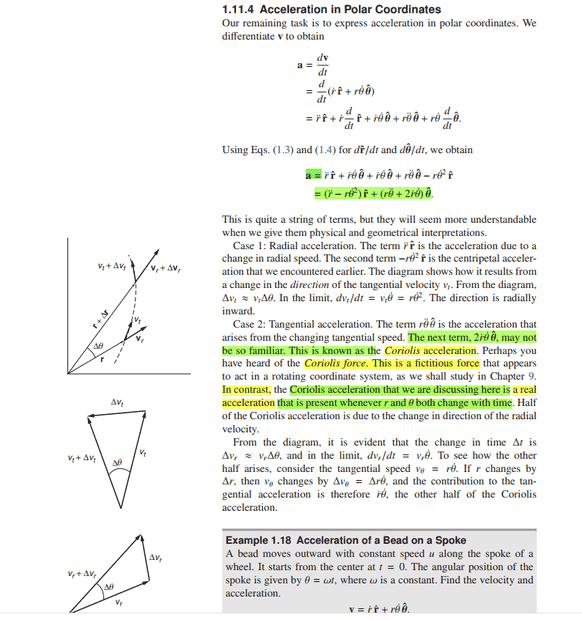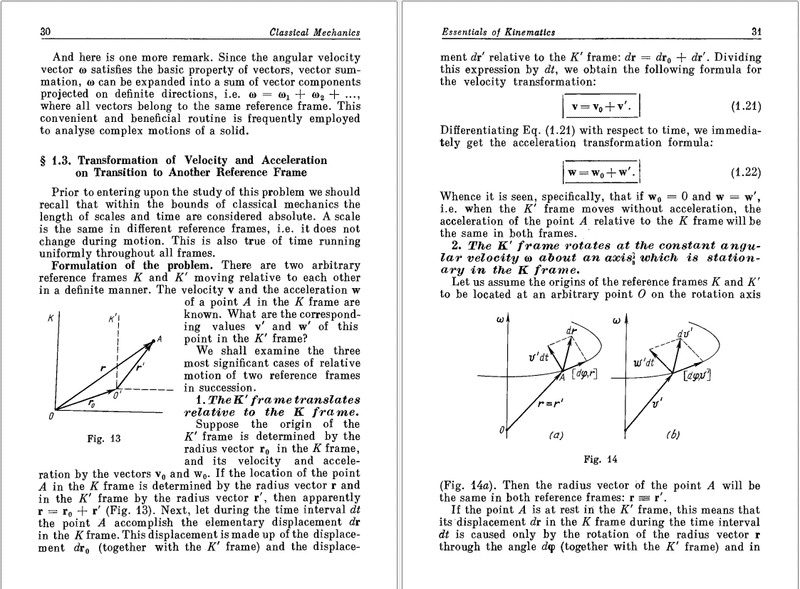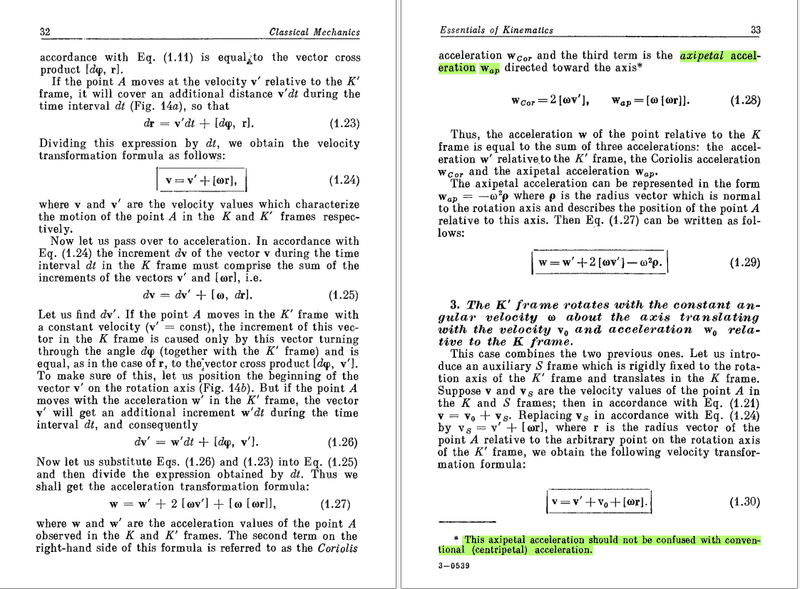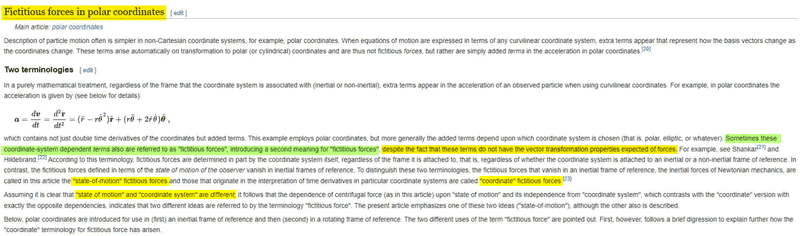# Weird Thing About Coriolis Acceleration and Coriolis Force?

NTesla
Homework Statement:
In Kleppner and Kolenkow's book: An Introduction to Mechanics, on page 34 (pasted below) on the topic titled "Acceleration in Polar coordinates", it has been mentioned that: "when ##r## and ##\theta## both change, then Coriolis acceleration acts which is ##real## and is "##In## ## contrast ##" to the Coriolis force which acts in a rotating frame of reference. "

What I'm trying to understand is that:
(1) If we are analyzing the situation using polar coordinate system, then if ##r## and ##\theta## both are changing, then Coriolis acceleration that acts (which by the way is real according to Kleppner and Kolenkow), is this Coriolis acceleration different from the Coriolis acceleration that would come into play due to Coriolis force, if we analyse the same situation from a rotating frame of reference ?

(2) Can Coriolis acceleration (real one) and Coriolis acceleration (due to Coriolis force when seen from rotating frame of reference) act simultaneously at a moving body(whose ##r## and ##\theta## both are changing with time), if we observe from a rotating frame of reference using polar coordinate system ?
Relevant Equations:
$$\overrightarrow{a} = (\ddot{r}-\dot{\theta}^{2}r)\hat{r}+(\ddot{\theta}r+2\dot{r}\dot{\theta})\hat{\theta}$$Homework Helper
Gold Member
2022 Award
I'm not sure your textbook's use of the term Coriolis acceleration is standard. As far as I can find online, it means the apparent acceleration resulting from the Coriolis force. The term referred to in the expression for acceleration in polar coordinates I would have called the Coriolis term.

••berkeman and vanhees71
NTesla
For the sake of discussion, even if we name it differently, would it act differently. When we are analyzing the situation from polar coordinate system in an inertial frame of reference, and if ##r## and ##\theta## both are changing, the expression for ##\vec{a}## does have a term(real NOT pseudo) which has the same magnitude and direction as Coriolis acceleration (from Coriolis force which arises when we are in non-inertial frame). But we are analyzing the situation from inertial frame. How is that fact to be reconciled with the pseudo Coriolis acceleration..? Will both be acting simultaneously if we are analyzing from non-inertial frame using polar coordinate system ?

Last edited:
•Nitin79
Homework Helper
For the sake of discussion, even if we name it differently, would it act differently. When we are analyzing the situation from polar coordinate system in an inertial frame of reference, and if ##r## and ##\theta## both are changing, the expression for ##\vec{a}## does have a term(real NOT pseudo) which has the same magnitude and direction as Coriolis acceleration (from Coriolis force which arises when we are in non-inertial frame). But we are analyzing the situation from inertial frame. How is that fact to be reconciled with the pseudo Coriolis acceleration..? Will both be acting simultaneously if we are analyzing from non-inertial frame using polar coordinate system ?
What is the distinction between a "real" term and a "pseudo" term?

Clearly the strokes that we make on pieces of paper when doing algebra and analytic geometry have no effect on what I would call the "real" world.

•vanhees71
NTesla
What is the distinction between a "real" term and a "pseudo" term?

Clearly the strokes that we make on pieces of paper when doing algebra and analytic geometry have no effect on what I would call the "real" world.
Could you kindly elaborate on what you meant to write.

Homework Helper
Could you kindly elaborate on what you meant to write.
When we do mathematics, we make marks on pieces of paper.
When we do physics, we associate observations about the real world with marks on pieces of paper.
None of that affects the path of a ball that has been struck by a bat.

What I consider "real" is what the ball does.
What I consider "pseudo" has to do with the marks on pieces of paper.

Some people would bring the distinction between "real" and "pseudo" onto the paper. When multiple descriptions of the same physical situation use different numbers for a thing (coordinate relative values), those things are "pseudo". When multiple descriptions of the same physical situation use the same numbers for the same thing (invariant values), those things are "real".

But that is what I mean when I use the words "real" and "pseudo". I do not know what you mean when you use the words "real" and "pseudo". You do not answer when asked.

Last edited:
•berkeman
NTesla
When we do mathematics, we make marks on pieces of paper.
When we do physics, we associate observations about the real world with marks on pieces of paper.
None of that affects the path of a ball that has been struck by a bat.

What I consider "real" is what the ball does.
What I consider "pseudo" has to do with the marks on pieces of paper.

Some people would bring the distinction between "real" and "pseudo" onto the paper. When multiple descriptions of the same physical situation use different numbers for a thing (coordinate relative values), those things are "pseudo". When multiple descriptions of the same physical situation use the same numbers for the same thing (invariant values), those things are "real".

But that is what I mean when I use the words "real" and "pseudo". I do not know what you mean when you use the words "real" and "pseudo". You do not answer when asked.
When you had asked the distinction between "real" and "pseudo" term, I felt that that was a rhetorical question. Do you think, that a person who is studying Coriolis force/acceleration is unaware of what the term real and pseudo mean..? I just hope that you have gone through the original question that I had posted. Had I asked what is Coriolis acceleration/force..? I would suggest you to go through my original question again, in case you haven't.

Homework Helper
When you had asked the distinction between "real" and "pseudo" term, I felt that that was a rhetorical question.

You'd used the phrase "term, (real, not pseudo)". I expected you to be able to explain what you meant when you used that phrase.

Are you going to answer that question now that I have clarified that is was sincere and not rhetorical.
I would suggest you to go through my original question again, in case you haven't.
Not going to do that for someone who cannot be bothered to answer a direct question and but instead complains about the responses that he is given.
And when I had replied: "Could you kindly elaborate on what you meant to write. ", what I had hoped for was that maybe you had an insight about the distinction between Coriolis acceleration(in polar coordinates) and Coriolis acceleration(due to rotating frame of reference).
If you had asked for such an insight, I might have responded as you'd hoped. But I'd already written what I meant to write. Asking for clarification of something that was already pretty clear sounded like a back-handed insult.
I answered the question you asked of me: "Could you kindly elaborate on what you mean to write".

Mentor

Mentor
After some thread cleanup, it is reopened provisionally. @NTesla please explain what you mean when you use the terms "real" and "pseudo" so that this thread can move forward. Thank you.

NTesla
It's Kleppner and Kolenkow's aforementioned observation regarding Coriolis acceleration(in polar coordinate) being real and different from Coriolis acceleration in rotating frame of reference, which should be the reason/point of discussion.

@berkeman, does the observation of Klepner and Kolenkow, as I have highlighted in the original post make sense to you..? Does it really depend upon how I define real and pseudo forces ? That would mean that what Kleppner and Kolenkow have written is right and the mistake lies in my understanding of the terms real and pseudo. As @haruspex has mentioned in his comment above that the textbook's use of those terms is not "standard", by which I am assuming that he said the textbook's use of those terms is not "right" and is the source of my confusion regarding Coriolis acceleration. You have approved of haruspex's comment above, which would mean that you agree with his notion that the book's usage of those terms is not standard or right. What I do not understand is that how come now, it has been presumed by people in this thread that the source of my original question depends on how I personally define the terms real and pseudo, and that the book's statements are right after all.

For anyone reading this thread and willing to make an effort to understand what I had originally asked, and for the sake of taking this discussion forward, if it at all depends on my understanding of the terms real and pseudo, which I don't think it does, here's how I define real and pseudo: Any phenomena which happens in nature is real, and it can be experienced by our physiological senses, and can be experimented with, and empirically recorded for study, follows the rigors of scientific study and for which we base/modify our scientific study to match the observed natural phenomena.

The term pseudo is used to explain something about which the rigors of scientific method do not apply or no previously understood concepts work. For example, when someone says pseudoscience, for a branch of study, then what he/she means to say is that in his opinion, and to the extent of his understanding, he is not able to find the justification presented to explain the present phenomena as scientific, reasonable or effective, and to some degree is skeptical of the study being scientific at all. Similarly, for pseudo medicine, what someone saying it means is that the medicine that is purported to be effective and is based on science, is not so. In the context of Physics, pseudo means an entity(for lack of any better term) such as pseudo force, which does not actually act on the body, but is required to explain a phenomena of movement of a body in non-inertial frame of reference, for which the existing concepts which work in inertial frame of reference, do not work.

Though, I now have given up hope that anyone here will be able to give any further meaningful comments regarding the original question.

Last edited:
NTesla
The original question is clear enough in this regard. So, there is no sense in asking for it separately again.

Last edited:
•jbriggs444
NTesla
I found the answer on wikipedia for my original question that makes atleast some sense (https://en.wikipedia.org/wiki/Mechanics_of_planar_particle_motion). I'm posting this with the thought that if someone in future comes with the same doubt as in the original question that i have posted, then their time and effort is not wasted by useless discussion about the definition of what the terms real and pseudo even mean.

Homework Helper
Gold Member
Here are some scribblings. I considered a mass moving at constant velocity ##\mathbf{v}##, parallel to the y-axis at constant distance ##x## from it. In Cartesian coordinates the position vector is $$\mathbf{r}=x~\mathbf{\hat x}+vt~\mathbf{\hat y}.$$We have polar unit vectors $$\mathbf{\hat r}=\frac{\mathbf{r}}{\|r\|}=\frac{x~\mathbf{\hat x}+vt~\mathbf{\hat y}}{\left[x^2+v^2t^2\right]^{1/2}}~;~~\mathbf{\hat \theta}=\frac{-vt~\mathbf{\hat x}+x~\mathbf{\hat y}}{\left[x^2+v^2t^2\right]^{1/2}}.$$ For the radial derivatives $$\dot r=\frac{v^2t}{\left[x^2+v^2t^2\right]^{1/2}}~;~~\ddot r=\frac{v^2 x^2}{\left[x^2+v^2t^2\right]^{3/2}}$$For the angular derivatives with ##\tan\theta = vt/x##,\begin{align} & \frac{v}{x}=\frac{d}{dt}(\tan\theta)=(1+\tan^2\theta)\dot\theta\implies \dot\theta=\frac{vx}{x^2+v^2t^2} \nonumber \\ & \ddot\theta=-\frac{2tv^3x}{\left[x^2+v^2t^2\right]^2}=-2\dot r\frac{vx}{\left[x^2+v^2t^2\right]^{3/2}} \nonumber \end{align} We can now assemble the acceleration terms a la Kleppner & Koleshnikow.

##a_r=\ddot r=\dfrac{v^2x^2}{\left[x^2+v^2t^2\right]^{3/2}}.##

Centripetal
##a_c=-r\dot {\theta}^2=-\left[x^2+v^2t^2\right]^{1/2}\left(\dfrac{vx}{x^2+v^2t^2}\right)^2=-\dfrac{v^2x^2}{\left[x^2+v^2t^2\right]^{3/2}}.##

Tangential
##a_t=r\ddot\theta = \left[x^2+v^2t^2\right]^{1/2}\left(-2\dot r\dfrac{vx}{\left[x^2+v^2t^2\right]^{3/2}}\right)=-\dfrac{2\dot r v x}{x^2+v^2t^2}.##

Coriolis
##a_{cor}=2\dot r \dot \theta=2\dot r \left(\dfrac{vx}{x^2+v^2t^2}\right )=\dfrac{2\dot r v x}{x^2+v^2t^2}.##

We see that no term is zero but the sums of the terms in the radial and tangential directions are zero. Given non-zero numerical values for ##v## and ##x##, one can calculate each of the four terms as a function of time. It is comforting that the sum of the radial parts and the sum of the tangential parts are both zero at all times.

Also note that if one sets ##x=0##, all four terms vanish identically as does the particle's angular momentum about the origin. It seems to me that we need to treat the four acceleration components the same way we treat angular momentum and torque. We cannot talk them without specifying the point about which they are to be considered. Furthermore, we see from this example that a rotating frame is not necessary for the Coriolis term to be non-zero.

So my answer to the question "does the Coriolis term exist in this example?" would be "Yes, because it can be calculated and a number for it can be found. Its effect upon the mass is not seen because it is canceled by an equal and opposite tangential term. The fact that this cancelation occurs does not make the Coriolis and tangential terms non-existent. Compare with similar reasoning in the case of a book at rest on a table top. The fact that the normal force exactly cancels the weight, does not make these two forces non-existent.

OK it exists, but is it real? Now we are getting philosophical. I can see @jbriggs444's argument
What I consider "real" is what the ball does.
What I consider "pseudo" has to do with the marks on pieces of paper.
and sidestep the issue by saying that these acceleration terms are as real as others that exist and depend on the choice of coordinates, e.g. angular momentum and torque.

NTesla
Thank you for such detailed reply..

I was going through I.E.Irodov's book: Fundamental laws of mechanics. On last page of the attachment(relevant pages of the book are attached below) it is mentioned that in equation 1.27 on page 32: $$\overrightarrow{a} = \overrightarrow{a^{'}}+2(\overrightarrow{w} \times \overrightarrow{v^{'}}) + (\overrightarrow{w}\times (\overrightarrow{w}\times\overrightarrow{r}))$$
that the third term is called axipetal acceleration, about which it is also mentioned that at the bottom of page 33 that it should not be confused with the conventional (centripetal) acceleration.

What I could understand after reading these pages is that even in inertial frame of reference(the K frame according to the pages pasted below), the expression for acceleration radially towards the centre of the curve that the particle is transiting is called axipetal but NOT centripetal.

So now the question is, how is the expression of centripetal acceleration derived, if it's different from the axipetal acceleration..? Up untill now, i used to think that the expression that has been described here as axipetal acceleration is the centripetal acceleration, but now this book cautions that these two are different.

How is it different and if it is different then how does one get the expression for the centripetal acceleration. ?

I have been searching the internet for answer, but even after having searched for days, I couldn't find any answer to the above question...Last edited:
Homework Helper
Gold Member
First of all your equation from Irodov is confusing in that all vectors in it are primed which does not make sense. It's a good thing you also posted the section of the book in which it appears as (1.27). Secondly, I assume you realize that the terms in (1.27) are similar to but not the same as the terms in K&K. Equation (1.27) is a transformation equation expressing the acceleration ##\vec{a}## in an inertial frame as seen by an observer in a frame rotating with angular velocity ##\vec{\omega}##. In a K&K you have the acceleration in a non-rotating frame expressed in polar coordinates instead of Cartesian. Now for your questions.

The standard derivation of the centripetal acceleration considers an object in uniform circular motion of radius ##R## about a point with constant angular velocity ##\vec{\omega}.## Then \begin{align} &\vec r=R\cos\omega t~\hat x+R\sin\omega t~\hat y \nonumber \\ & \vec a= \frac{d^2\vec r}{dt^2}=-\omega^2 R\cos\omega t~\hat x - \omega^2 R\sin\omega t~\hat y=-\omega^2 \vec r. \nonumber \end{align}Note that the position vector of the object is in the plane of the circle that the object describes and the acceleration points towards that center. In this case then, ##\vec r## and ##\vec \omega## are perpendicular. Then $$\vec \omega \times( \vec \omega \times \vec r)=\cancel{\vec{\omega}(\vec {\omega}\cdot \vec r)}-\vec r(\vec {\omega} \cdot \vec{\omega})=-\omega^2 \vec r.$$Irodov makes a distinction between centripetal and axipetal that one does not usually see in textbooks. Consider an object on the surface of the Earth rotating with it. If the object is on the Equator, its acceleration is centripetal because it points towards the center of the Earth (origin) and its position vector is in the plane of the circle it describes. If the object is at some non-zero latitude, then its position vector is not in the plane of the circle it describes and ##-\omega^2 \vec r## points towards the axis of rotation but not the origin, hence the acceleration should be called (according to Irodov) axipetal. Other textbooks call it centripetal with the understanding that the term points towards the center of a circle at fixed latitude.

Summary on edit
The term is ##\vec a_c=\vec \omega \times( \vec \omega \times \vec r).## Its magnitude is ##a_c= \omega^2 ~r~\sin\theta## where ##\theta## is the angle between ##\vec\omega## and ##\vec r.## Its direction is perpendicular to the plane formed by ##\vec v~ (= \vec \omega \times \vec r)## and ##\vec \omega##. Then the position vector ##\vec r## has constant magnitude and the object describes a circle of radius ##r\sin\theta## the plane of which is perpendicular to ##\vec \omega.## It is what it is and what you call it does not really matter.

Last edited:
•NTesla
NTesla
Only the vectors in ##K^{'}## frame of reference(which is a rotating frame of reference) have a prime attached to them. And yes, I'm aware of the second point that you've made. I agree with you there..

Thank you for the detailed explanation.. It's very helpful.

Last edited:
NTesla
OK it exists, but is it real?
From the wikipedia page that I had posted in post#13, I'm posting the relevant part here below. The part which is bothering me now is the line written in the image below: "Sometimes these coordinate-system dependent terms (in the expression for acceleration in polar coordinates(inertial frame of reference)) are also referred to as as "fictitious forces", introducing a second meaning for "fictitious forces" despite the fact that these terms do not have the vector transformation properties expected of forces".

How come the vector transformation properties are not applicable to the coordinate-system dependent "fictitous forces" (which by the way are real acceleration/forces according to Kleppner and Kolenkow)?Homework Helper
Gold Member
How come the vector transformation properties are not applicable to the coordinate-system dependent "fictitous forces" (which by the way are real acceleration/forces according to Kleppner and Kolenkow)?
Because they are cross products which are pseudovectors that do not have the same transformation properties as polar vectors. BTW, "pseudo" in this context means "fake" not "unreal" or "non-existent".

•NTesla
Gold Member
2022 Award
I'd rather call the axial vs. polar vectors. "Pseudo" is rarely a good ingredient for a name ;-)).

Homework Helper
Gold Member
I'd rather call the axial vs. polar vectors. "Pseudo" is rarely a good ingredient for a name ;-)).
I agree, but I conformed with the title of the Wikipedia article to avoid further confusion.

•vanhees71
Homework Helper
Gold Member
2022 Award
Because they are cross products which are pseudovectors that do not have the same transformation properties as polar vectors. BTW, "pseudo" in this context means "fake" not "unreal" or "non-existent".
Am I right in thinking that the cross product of two polar vectors or two axial vectors is axial, but the cross product of one of each is polar?

•vanhees71
Homework Helper
Gold Member
That's what the Wikipedia article says and I believe it's correct.

Homework Helper
Gold Member
2022 Award
•vanhees71 and Callumnc1
Homework Helper
Gold Member
•vanhees71 and Callumnc1
Gold Member
2022 Award
Am I right in thinking that the cross product of two polar vectors or two axial vectors is axial, but the cross product of one of each is polar?
That's easy to prove. A polar vector ##\vec{p}## transforms as ##\vec{p} \rightarrow -\vec{p}## and an axial vector ##\vec{a}## as ##\vec{a} \rightarrow +\vec{a}## (EDIT: Typo corrected in view of #28) under space reflections, which implies that for two polar vectors
$$\vec{p}_1 \times \vec{p}_2 \rightarrow (-\vec{p}_1) \times (-\vec{p}_2) = + \vec{p}_1 \times \vec{p}_2,$$
i.e., ##\vec{p}_1 \times \vec{p}_2## is an axial vector. In the same way you find that the cross product of two axial vectors transforms a again as an axial vector, and the cross product of a polar and an axial vector transform as a polar vector.

In this connection one may note that there are also scalars and pseudo-scalars (here I've to use "pseudo", because I've never seen another name for it ;-)): The scalar product of two polar or two axial vectors obviously are invariant under space reflections ("scalars") while the scalar product of a polar and an axial vector flips its sign under space reflections ("pseudo-scalars").

Last edited:
Just my 2 cents: it seems like the author calls an active coordinate transformation resulting in an acceleration a "real" acceleration, and associates the origin of inertial forces with passive transformations (a change of coordinate system).

"Real" as in "The result of a force acting on the object" in line with Newton's second law. In the passive point of view the force acts on the object defining the coordinate system.

•vanhees71
Homework Helper
Gold Member
##\dots~## and an axial vector ##\vec{a}## as ##\vec{a} \rightarrow -\vec{a}## under space reflections ##~\dots##
Did you mean "##\dots~## and an axial vector ##\vec{a}## as ##\vec{a} \rightarrow +\vec{a}## under space reflections ##~\dots##"?

•vanhees71